Courses

# Test: Subtraction- 1

## 10 Questions MCQ Test Mathematics for Class 2 | Test: Subtraction- 1

Description
This mock test of Test: Subtraction- 1 for Class 2 helps you for every Class 2 entrance exam. This contains 10 Multiple Choice Questions for Class 2 Test: Subtraction- 1 (mcq) to study with solutions a complete question bank. The solved questions answers in this Test: Subtraction- 1 quiz give you a good mix of easy questions and tough questions. Class 2 students definitely take this Test: Subtraction- 1 exercise for a better result in the exam. You can find other Test: Subtraction- 1 extra questions, long questions & short questions for Class 2 on EduRev as well by searching above.
QUESTION: 1

### Directions: Find the missing number. 63 - 54 = ____

Solution: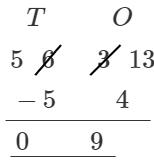QUESTION: 2

### Directions: Find the missing number. 27 - ____ = 22

Solution: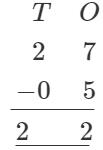QUESTION: 3

### 43 - ____ = 22

Solution: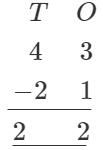QUESTION: 4

How much should be subtracted from 61 to get 29.

Solution:QUESTION: 5

Raghu had 41 mangoes. Out of 41. 12 mangoes were rotten. How many fresh mangoes Raghu has?

Solution: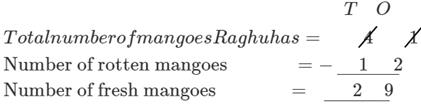QUESTION: 6

Directions: Answer the following questions value of a = 19. b = 43 and c = 15
Fill the correct value in the blank. a + b = ? - c?

Solution:

a + b = 62, c = 15 So,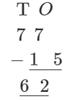QUESTION: 7

Amandeep is reading a story book that has 78 pages. To complete the book. Amandeep has to read 23 more pages. How many pages has Amandeep read so far?

Solution:

Total number of pages = 78
Number of pages left to read = 23

QUESTION: 8

The next number in the series is 34, 29, 24, ______

Solution:

The difference between numbers is 5.
So, the next number is 24 - 5 = 19.

QUESTION: 9

Find the missing ones digit. 5____- 35 = 21

Solution: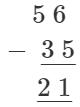QUESTION: 10

Roman's mother asked him to bring a packet of butter of Rs. 21 from a nearby shop. After reaching to the shop. he came to know that he had only Rs. 15 So. he started crying, thinking that his mother will scold him. As a friend how would you help Roman?

Solution:

We should always give right advise to our friends.